# Z-Source Inverter for Energy Management and Vector Control for Electric Vehicle Based PMSM

Z-Source Inverter for Energy Management and Vector Control for Electric Vehicle Based PMSM
Laboratory of L2GEGI, Department of Electrical Engineering, Faculty of Sciences Applied, University of Tiaret, Tiaret 14000, Algeria

Dipartimento di Automazione, Università degli Studi di Cassino, Cassino 03043, Italy

Corresponding Author Email:
Page:
883-892
|
DOI:
https://doi.org/10.18280/jesa.530614
25 July 2020
|
Accepted:
8 October 2020
|
Published:
23 December 2020
| Citation

OPEN ACCESS

Abstract:

Electric vehicles have gained considerable attention recently due to the ever increasing demand for a viable alternative to the current fossil fuel-dependent modes of transportation. These automobiles are reliant on power electronics to generate the energy required for the motor. Traditional converters, namely the V-source (VS) and C-source (CS), are vulnerable to EMI noise, their main circuits cannot be interchangeable and they are either a boost or a buck converter. Therefore, their output voltage is strictly higher or lower than the input voltage. In an effort to negate these drawbacks, new inverters such as the Z-source were conceptualized. This work aims to study the applicability of the Z-source in the traction chain of an electric vehicle in order to feed a permanent magnet synchronous motor (PMSM). The latter is controlled with field oriented vector control reinforced with a backstepping technique in an attempt to ensure tracking ability and robustness. Energy management is also supported in this article in an effort to optimize the performance of the electric vehicle under different operating conditions. The simulation results show the effectiveness of the proposed system in enhancing the energy management of the vehicle, in addition to its simplicity which can facilitate an eventual implementation using a DSP or a Dspace platform.

Keywords:

battery, electric vehicle control, energy management, fuel cell, permanent magnet synchronous motor, backstepping control, vector control

1. Introduction

Power electronics have been utilized in a wide spectrum of applications, from doing a fly-back converter as grid interference topology in renewable energy , to designing low voltage DC systems in house appliances , aerospace applications , and most notably their integration into the traction chain of electric vehicles . In order to achieve the latter, many research efforts have been dedicated to the development of new inverters such as Z-source inverters , T-type inverters and flying capacitor inverters [6, 7] that are adapted to electric vehicles .

Z-source has become a very promising topology for vehicular applications due its various features mainly the ability to be open- or short-circuited which provides a mechanism for the main converter circuit to step up or step down the voltage as desired . In addition to that, the output AC has a wide range of obtainable voltages regardless of the DC input. These features ensure that the Z-source inverter offers high reliability against electromagnetic interference (EMI), as well as reducing the number of components and the cost to achieve the same function as a conventional two-stage inverter (buck-boost inverter). The configuration allows the elimination of dead time, which enhances the quality of the output current/voltage waveform (i.e., no distortion).

For the EV power supply, the fuel cell (FC) is considered as the main power source. However, under low load conditions the fuel cell (FC) becomes insufficient, so the use of a battery as a back-up source is necessary to avoid limited driving range and also to prolong the fuel cell’s lifetime. Therefore, the power management is adapted to assist the energy flow in the traction chain components with the proper choice of the motor.

Permanent Magnet Synchronous Motor (PMSM) is one of the electric motors that are often used as electric vehicle drives due to several reasons including compact structures, high air-gap flux density, high power density, high torque to inertia ratio, and high efficiency . The PMSM requires a robust control strategy, that is to say it stands in need of fast and accurate response, quick recovery from any disturbances and insensitivity to parameter variations. On this account, many modern control methods, such as the backstepping control have been urged in order to improve the PMSM’s performances .

The backstepping control is an efficient method for nonlinear systems; it is a new type recursive and systematic methodology for the feedback control for systems with matched uncertainties. The major feature of this technique is the use of virtual variable to simplify high order systems; thus, making it possible to gradually derive the final outputs through suitable Lyapunov functions .

This paper is structured as follow: The description of the traction chain components is discussed is Section.2. the Section.3 is dedicated to the Z-source inverter; along with its operating modes. Section 4 deals with the modelling and control of the PMSM. Section.5 discusses the energy management technique. The simulation results of the study are shown in Section.6. Section.7 summarizes the work done in the conclusion.

2. Description of the Traction Chain

The schema illustrated in Figure 1 demonstrates the components of the electric traction system based electric vehicle. The fundamental objective of the proposed design is the control of the speed using backstepping control.

The constituents are made up of: a battery supplied DC voltage source, a fuel cell, a MOSFET based Z-source inverter, a field oriented control applied on two permanent magnet synchronous motors located in the rear of the electric vehicle connected to the two wheels. The performance results of each of the elements will be observed in order to interpret the effect of control technique [13, 14].

## 1.pngFigure 1. The traction chain components

3. Z-source Inverter

3.1 Description and modeling of the Z-source inverter

The Figure 2 shows basic topology of the ZSI, which looks like a lattice network containing two inductors (L1 and L2) and two capacitors (c1 and c2) connected in X shape to couple the inverter to DC voltage source, the latter can be a battery, fuel cell, photovoltaic array, diode rectifier, thyristor converter, inductor, capacitor or combination of inductor and capacitor. The full bridge consists of two legs, each of which contains two mutually complementary switches. Thus, the load will be continuously supplied by the output current and the voltage is exclusively imposed by the state of the switch .

## 2.png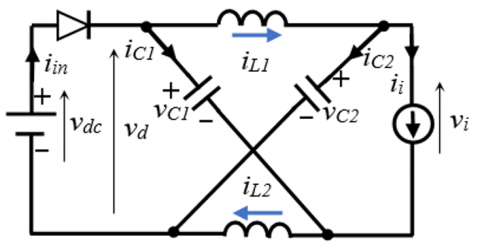Figure 2. Equivalent circuit of the impedance network

In steady state, the modelling of the Z-source inverter can be expressed as follow [16, 17]:

During the time interval T0:

${{v}_{L1}}={{v}_{L2}}={{v}_{L}}={{v}_{C1}}={{v}_{C2}}={{v}_{C}}$     (1)

${{v}_{d}}={{v}_{L}}+{{v}_{C}}=2{{v}_{C}}$     (2)

${{v}_{i}}=0$      (3)

During time interval T1:

${{v}_{L}}={{v}_{dc}}-{{v}_{C}}$     (4)

${{v}_{d}}={{v}_{dc}}$     (5)

${{v}_{i}}={{v}_{C}}-{{v}_{L}}=2{{v}_{C}}-{{v}_{dc}}$      (6)

The average voltage of the inductors over one switching period (T) should be zero in steady state and $T={{T}_{0}}+{{T}_{1}}$ giving:

${{v}_{c}}=\frac{{{T}_{1}}}{{{T}_{1}}-{{T}_{0}}}{{v}_{dc}}$     (7)

Using the above mentioned equations, the peak DC voltage ${{\hat{v}}_{i}}$ and the peak AC output voltage ${{\hat{v}}_{i}}$ can be written as:

${{\hat{v}}_{i}}=2{{v}_{c}}-{{v}_{dc}}=\frac{1}{1-\frac{2{{T}_{0}}}{T}}{{v}_{dc}}=B{{v}_{dc}}$    (8)

${{\hat{v}}_{x}}=M\frac{{{{\hat{v}}}_{i}}}{2}=B\frac{M\text{ }{{v}_{dc}}}{2}$      (9)

It’s obvious in Eq. (9) that the AC output voltage is boosted by the factor B, thus the use of a boost (DC-DC) converter is no longer needed. In a view of its special structure, the ZSI has an extra switching state, when the load terminals are shorted through both the upper and lower switching devices of any phase leg, which is called the shoot-through (ST) state besides the eight traditional non-shoot through (NST) states. This switching state provides the unique buck-boost feature to the inverter. The Table 1 shows how the shoot-through state of a three-phase leg Z-source can be controlled .

Table 1. Switching states of three phase ZSI.

(!S1, !S2 and !S3 are the complements of S1, S2 and S3 respectively)

 Switching states S1 S2 S3 S4 S5 S6 (V) Active states 1 0 0 0 1 1 Finite 1 1 0 0 0 1 0 1 0 1 0 1 0 1 1 1 0 0 0 0 1 1 1 0 1 0 1 0 1 0 Zero states 0 0 0 1 1 1 Zero 1 1 1 0 0 0 ST states 1 S2 S3 1 !S2 !S3 Zero S1 1 S3 !S1 1 !S3 S1 S2 1 !S1 !S2 1 1 1 S3 1 1 !S3 1 S2 1 1 !S2 1 S1 1 1 !S1 1 1 1 1 1 1 1 1

## 3.png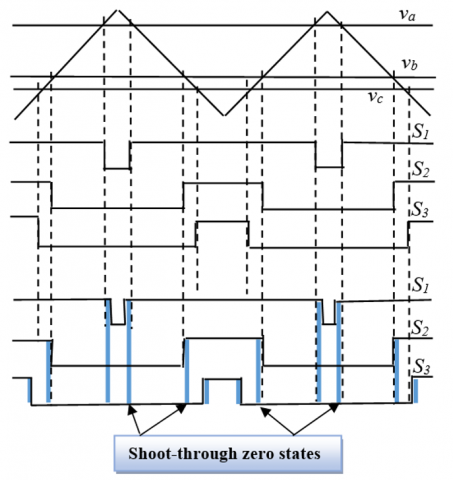Figure 3. The difference between a traditional carrier-based PWM and a ST PWM

The control strategy applied to the Z-source inverter is a pulse width modulation (PWM), its principle consists of inserting the Shoot-through states at each transition by overlapping the upper and lower conductor signals, which can be derived by a suitable level by shifting the modulation signals of the VSI (Voltage Source Inverter) . The offset values are adjusted correctly to ensure that the occupancy time of the two zero states is identical. The characteristic of this modulation strategy, shown in Figure 3; is that the transition time in a switching cycle is the similar to that of the Voltage Source Inverter (VSI), the "Shoot-Through ST" state is divided into six parts and the equivalent switching frequency of the impedance network is six times the switching frequency. Therefore, the volume of the inductors could be considerably reduced [18, 19].

3.2 Operating modes

The general operation of a ZSI can be illustrated by simplifying the AC side circuit and the D input diode with two switches.

As already discussed, the Z-source inverter uses the shoot-through zero states to increase the voltage in addition to the traditional 6 active states and 2 zero states . Assuming that the inductor current is almost constant; when the inductance is low, the inductor current can become very rippled or even discontinuous. Instead of having the two operating modes [21, 22], the Z-source inverter can have five different operating modes.

Mode 1: shown in Figure 4(a), the inverter bridge is operating in a shoot-through zero state. During this mode the bridge can be viewed as an open-circuit, the DC voltage appears across the ‘inductor and the capacitor’, except that no current flows to the load, from the DC source.

The voltages across the capacitors are:

${{v}_{L1}}={{v}_{C1}},{{v}_{L2}}={{v}_{C2}}$      (10)

Assuming that the inductors L1 and L2 and capacitors C1 and C2 have the same value, respectively. The voltage equations of the ZSI can be written as:

$\left\{ \begin{matrix} {{v}_{L1}}={{v}_{L2}}={{v}_{L}} \\ {{v}_{C1}}={{v}_{C2}}={{v}_{C}} \\\end{matrix} \right.$     (11)

The inductor current increases linearly with the assumption that the capacitor voltage is constant during this period.

${{i}_{L1}}={{i}_{L2}}={{i}_{L}}$     (12)

Mode 2: The inverter is in a non-shoot through state (one of the eight conventional states), In this mode according to Figure 4(b), the DC source voltage is linked to the inductor and the capacitor allowing the energy flow to the load via the inductor. The inductor discharges in this mode; its voltage is given by the following equation.

${{v}_{L}}={{v}_{0}}-{{v}_{C}}$     (13)

## 4.pngFigure 4. Operating modes of the Z-source inverter

The capacitor voltage is higher than that of the output which makes the inductor voltage negative, that leads to a linear decrease in the inductor current. This latter has to approve the following condition:

${{i}_{L}}>\frac{1}{2}{{i}_{i}}$     (14)

The input current from the DC source becomes:

${{i}_{in}}={{i}_{L1}}+{{i}_{C1}}={{i}_{L1}}+({{i}_{L2}}-{{i}_{i}})=2{{i}_{L}}-{{i}_{i}}>0$     (15)

Mode 3: Illustrated in Figure 4 (c), the inverter is in one of the 6 active states, and at the end of Mode 2, the inductor current decreases to half of the inverter DC side current ii. As a result, the input current becomes zero and the diode becomes reverse-biased. Supposing that the inductances L1 and L2 are negligeable, the inverter voltage and current are given as:

$\left\{ \begin{matrix} {{v}_{i}}={{v}_{c}} \\ {{i}_{i}}=2{{i}_{L}} \\\end{matrix} \right.$      (16)

Mode 4: In this mode, the ZSI is isolated from the load because the inverter current is equal to zero. Hence, the diode is blocked and the inverter is in an open-circuit, as shown in Figure 4 (d).

Mode 5: The Figure 4 (e) shows that the inverter is in an active state during this mode and enters a free-wheeling state owing to the fact that the inductor current is smaller than half of the inverter DC current. The free-wheeling diodes, shown in Figure 4, ensure that the inverter enters in a shoot-through state and all the equations of mode.1 hold true.

4. Modeling and Control of the PMSM

4.1 Modeling of the permanent magnet synchronous motor

The induction motor mathematical model in d-q coordinates established in a rotor flux-oriented reference frame can be written as :

\left\{ \begin{align}& {{v}_{sd}}={{R}_{s}}{{i}_{sd}}+\frac{d{{\psi }_{sd}}}{dt}-\omega {{\psi }_{sq}} \\& {{v}_{sq}}={{R}_{s}}{{i}_{sq}}+\frac{d{{\psi }_{sq}}}{dt}+\omega {{\psi }_{sd}} \\\end{align} \right.     (17)

The stator flux linkages are given by:

\left\{ \begin{align} & {{\psi }_{sd}}={{L}_{d}}{{i}_{sd}}+{{\psi }_{f}} \\ & {{\psi }_{sq}}={{L}_{q}}{{i}_{sq}} \\\end{align} \right.    (18)

The electromagnetic torque and the rotor speed are given by:

\left\{ \begin{align} & {{T}_{em}}=p{{\psi }_{F}}{{i}_{sq}}+p({{L}_{d}}-{{L}_{q}}){{i}_{sd}}{{i}_{sq}} \\ & \frac{d{{\omega }_{r}}}{dt}=\frac{p}{J}{{T}_{em}}-\frac{f}{J}{{\omega }_{r}}-\frac{p}{J}{{T}_{l}} \\\end{align} \right.     (19)

4.2 Nonlinear backstepping control

The main idea of this approach is to recursively design controllers without exceeding the system’s order, each step produces virtual control variable and force it to become a stabilizing function; Henceforth, balancing up the error variable by properly designing the related control input on the basis of Lyapunov stability theory .

The model of the PMSM can be written as follows [25-27]:

\left\{ \begin{align} & {{{\dot{x}}}_{1}}=-\frac{{{R}_{s}}}{{{L}_{d}}}{{x}_{1}}+\frac{{{L}_{q}}}{{{L}_{d}}}p{{x}_{2}}{{x}_{3}}+\frac{1}{{{L}_{d}}}{{v}_{d}} \\ & {{{\dot{x}}}_{2}}=\frac{p{{\psi }_{F}}}{J}{{x}_{3}}-\frac{f}{J}{{\omega }_{r}}-\frac{1}{J}{{T}_{l}} \\ & {{{\dot{x}}}_{3}}=-\frac{{{R}_{s}}}{{{L}_{d}}}{{x}_{3}}-\frac{{{L}_{d}}}{{{L}_{q}}}p{{x}_{2}}{{x}_{1}}-\frac{p}{{{L}_{q}}}{{\psi }_{F}}{{x}_{2}}+\frac{1}{{{L}_{q}}}{{v}_{q}} \\\end{align} \right.     (20)

where, x1=id, x2=wr, x3=iq, u1=vd, and u2=vq. The variables u1 and u2 are the actual control and x is the state vector.

The direct axis current id is always forced to be zero in order to orient all the linkage flux in the d-axis and achieve maximum torque per ampere variations corresponds to the variation of the reference torque at different times. The first regulated variable is:

${{z}_{1}}={{x}_{1}}$    (21)

The derivative of Eq. (21) can be calculated as:

${{\dot{z}}_{1}}=-\frac{{{R}_{s}}}{{{L}_{d}}}{{x}_{1}}+\frac{{{L}_{q}}}{{{L}_{d}}}p{{x}_{2}}{{x}_{3}}+\frac{1}{{{L}_{d}}}{{v}_{d}}$     (22)

The first Lyapunov candidate is chosen as:

${{V}_{1}}=\frac{1}{2}{{z}_{1}}^{2}$      (23)

The derivative of Eq. (23) can be calculated as:

${{\dot{V}}_{1}}={{z}_{1}}{{\dot{z}}_{1}}={{z}_{1}}\left( -\frac{{{R}_{s}}}{{{L}_{d}}}{{z}_{1}}+\frac{{{L}_{q}}}{{{L}_{d}}}p{{x}_{1}}{{x}_{2}}+\frac{1}{{{L}_{d}}}{{v}_{d}} \right)$      (24)

The direct axis voltage control input can be selected by:

${{v}_{d}}=-{{L}_{d}}\left( {{c}_{1}}{{z}_{1}}+\frac{{{L}_{q}}}{{{L}_{d}}}p{{x}_{2}}{{x}_{3}} \right)$    (25)

where, c1 is a positive design constant, so Eq. (22) becomes:

${{\dot{z}}_{1}}=-\left( {{c}_{1}}+\frac{{{R}_{s}}}{{{L}_{d}}}{{z}_{1}} \right)$      (26)

Therefore, Eq. (24) becomes:

${{\dot{V}}_{1}}=-\left( {{c}_{1}}+\frac{R}{{{L}_{d}}}{{z}_{1}}^{2} \right)$    (27)

The reference speed tracking is the main reason of this control design, so the next regulated variable is the speed.

${{z}_{2}}={{x}_{2}}-{{\omega }_{ref}}$      (28)

Its derivative is written as:

${{\dot{z}}_{2}}=\frac{p{{\psi }_{F}}}{J}{{x}_{3}}-\frac{f}{J}{{x}_{2}}-\frac{1}{J}{{T}_{l}}-{{\dot{\omega }}_{ref}}$      (29)

With wref is the reference speed, note that z3=x3-a, and a is the stabilizing function chosen as follows:

$\alpha =\frac{J}{p{{\psi }_{F}}}\left( \frac{f}{J}{{\omega }_{ref}}+\frac{1}{J}{{T}_{l}}+{{{\dot{\omega }}}_{ref}} \right)$      (30)

The Eq. (29) can be written as:

${{\dot{z}}_{2}}=-\frac{f}{J}{{z}_{2}}+\frac{p{{\psi }_{F}}}{J}{{z}_{3}}$      (31)

The second Lyapunov candidate is chosen as:

${{V}_{2}}=\frac{1}{2}{{c}_{2}}{{z}_{2}}^{2}$       (32)

where, c2 is a positive constant, so the derivative of Eq. (32) is computed as:

${{\dot{V}}_{2}}={{c}_{2}}{{z}_{2}}{{\dot{z}}_{2}}$       (33)

Eq. (33) can also be written as:

${{\dot{V}}_{2}}=-\frac{f}{J}{{c}_{2}}{{z}_{2}}^{2}+\frac{p{{\psi }_{F}}}{J}{{c}_{2}}{{z}_{2}}{{z}_{3}}$     (34)

The derivative of the error variable  ${{z}_{3}}$ is given as:

\begin{align} & {{z}_{3}}=-\frac{{{R}_{s}}}{{{L}_{q}}}{{x}_{3}}-\frac{{{L}_{d}}}{{{L}_{q}}}p{{x}_{2}}{{x}_{1}}-\frac{p}{{{L}_{q}}}{{\psi }_{F}}{{x}_{2}} \\ & +\frac{1}{{{L}_{q}}}{{v}_{q}}-\frac{J}{p{{\psi }_{F}}}\left( \frac{f}{J}{{{\dot{\omega }}}_{ref}}+{{{\ddot{\omega }}}_{ref}} \right) \\\end{align}      (35)

With the selection of the global Lyapunov function:

$V=\dot{V}_{1}+\dot{V}_{2}+z_{3} \dot{z}_{3}$    (36)

\begin{align} & V=-\left( {{c}_{1}}+\frac{{{R}_{s}}}{{{L}_{d}}} \right){{z}_{1}}^{2}-\frac{f}{J}{{c}_{2}}{{z}_{2}}^{2} \\ & +{{z}_{3}}[\frac{p{{\psi }_{F}}}{J}{{c}_{2}}{{z}_{2}}-\frac{{{R}_{s}}}{{{L}_{q}}}{{x}_{3}}-\frac{{{L}_{d}}}{{{L}_{q}}}p{{x}_{2}}{{x}_{1}}-\frac{p}{{{L}_{q}}}{{\psi }_{F}}{{x}_{2}} \\ & +\frac{1}{{{L}_{q}}}{{v}_{q}}-\frac{J}{p{{\psi }_{F}}}(\frac{f}{J}{{{\dot{\omega }}}_{ref}}+{{{\ddot{\omega }}}_{ref}})] \\\end{align}      (37)

## 5.pngFigure 5. The control scheme

Finally, in order to make the derivative of the complete Lyapunov function (21) be negative definite, the q-axis voltage control input is chosen as follows:

\begin{align} & V={{L}_{q}}[-\frac{p{{\psi }_{F}}}{J}{{c}_{2}}{{z}_{2}}+\frac{{{R}_{s}}}{{{L}_{q}}}{{x}_{3}}-\frac{{{L}_{d}}}{{{L}_{q}}}p{{x}_{2}}{{x}_{1}} \\ & +\frac{p}{{{L}_{q}}}{{\psi }_{F}}{{x}_{2}}+\frac{J}{p{{\psi }_{F}}}(\frac{f}{J}{{{\dot{\omega }}}_{ref}}+{{{\ddot{\omega }}}_{ref}})-{{c}_{3}}{{z}_{3}}] \\\end{align}     (38)

Thus, substituting (38) in (37) gives the following equation:

$\dot{V}=-\left( {{c}_{1}}+\frac{{{R}_{s}}}{{{L}_{d}}} \right){{z}_{1}}^{2}-\frac{f}{J}{{c}_{2}}{{z}_{2}}^{2}-{{c}_{3}}{{z}_{3}}$    (39)

From (37) and (39) one is positive and the other one is negative; this clearly means that the resulting closed-loop system is asymptotically stable. Furthermore, all the error variables z1, z2 and z3 will converge to zero asymptotically. The Figure 5 shows the control scheme.

5. Energy Management

An efficient energy management aims to make powertrain components operate in high efficacy while maintaining a satisfactory amount of energy in the storage devices. Moreover, the consumption of the fuel has to be minimized without effecting the vehicle performances, that can only be obtained by adapting an appropriate control strategy .

The Figure 6 displays the different driving modes along with the energy flow.

Mode1: Is the high power generation mode, in case of acceleration or uphill driving the energy demand is high, both of the fuel cell (FC) and the battery are providing energy to the motor.

${{P}_{load}}={{P}_{FC}}+{{P}_{bat}}$    (40)

Mode2: Known as medium power mode, the fuel cell (FC) is the only energy source in this mode, it can also provide energy to the battery if its state of charge (SOC) is less than 30%.

${{P}_{load}}={{P}_{FC}}$     (41)

Mode3: The low power mode, in this case the vehicle is run by the energy generated by the battery alone, the fuel cell is stopped because its system efficiency, including the energy consumption of the auxiliary parts, such as the air compressor, decreases in the low power consumption.

${{P}_{load}}={{P}_{bat}}$     (42)

Mode4: The regenerative braking mode, the Fuel cell is not generating any power; on the other hand, the battery absorbs the energy provided by the motor, which acts as a generator in this case.

${{P}_{load}}=-{{P}_{bat}}$    (43)

Note that there is another mode called the stop mode, where there is no energy flow between the components.

${{P}_{load}}={{P}_{FC}}={{P}_{bat}}=0$     (44)

The proposed chart of the power management system is described in Figure 7. It defines in the first place the different powers involved in our hybrid system; if the power supplied is greater than the requested power in this case the excess power will be stored in the battery. If the requested power is greater than that of the generated that leads us to the following two cases. If the state of charge is greater than 30% the storage devices are switched on. In the opposite case, the battery stops working, which forces the fuel cell to charge it.

## 6.png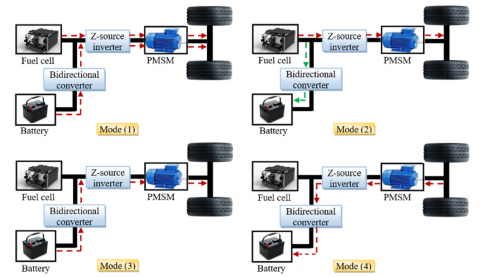Figure 6. The different modes of energy flow

## 7.png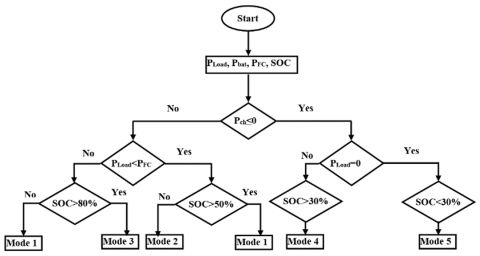Figure 7. The flowchart of the energy management controller

6. Simulation Results and Discussion

In an effort to validate the efficiency and dynamic performance of the proposed strategy, numerical simulations were performed using MATLAB/Simulink software under varying load conditions on an electric vehicle powered by a permanent magnet synchronous motor (PMSM), whose engine parameters are listed in Table 2 of the appendix. The battery and fuel cell parameters are also listed in Table 3 of the same appendix.

Starting with the acceleration pedal positions illustrated in Figure 8, which is considered as the input of the controlled system, in other words; it is the reference that all of the components are compelled to follow. Figures 9, 10 and 11 show the DC voltage, Fuel cell (FC) voltage and the fuel cell current respectively.

## 8.pngFigure 8. The acceleration pedal positions

## 9.png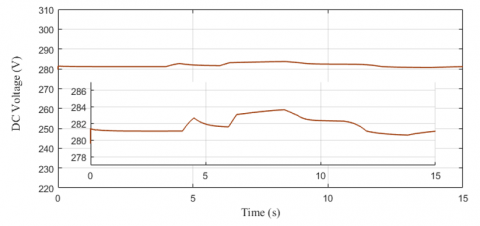Figure 9. The DC bus voltage

## 10.png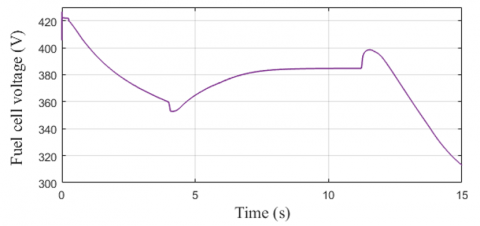Figure 10. The fuel cell voltage

## 11.png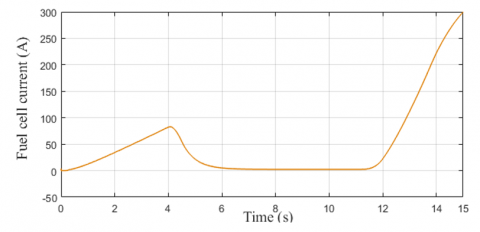Figure 11. The fuel cell current

## 12.png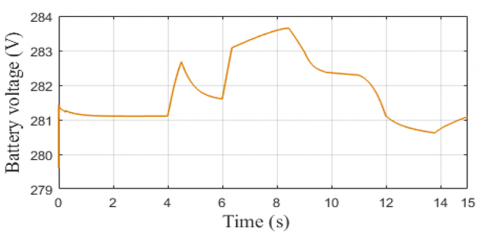Figure 12. The battery voltage

## 13.png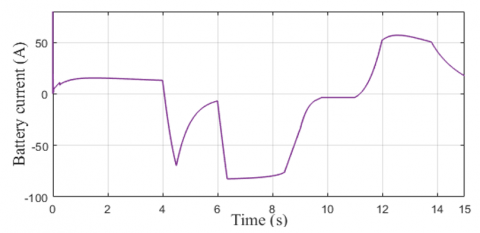Figure 13. The battery current

## 14.png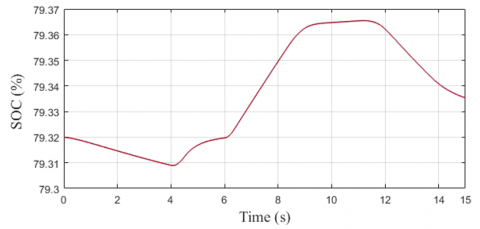Figure 14. The state of charge of the battery

## 15.png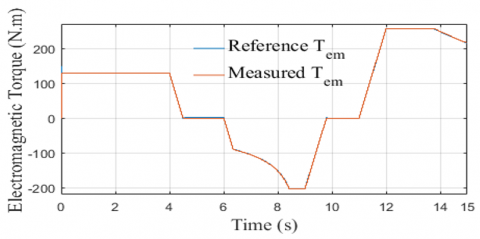Figure 15. The electromagnetic toque response

## 16.png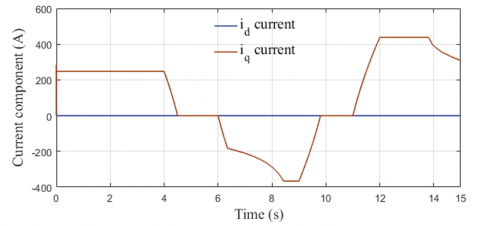Figure 16. The stator current in d-q coordinates

The Figures 12, 13 and 14 show the voltage, the current and the state of charge of the secondary source of energy.

The Figure 15 represents the dynamic of the electromagnetic torque, which is one of the key performance advantages of any type of vehicle, it can be considered as the ‘strength’ of the car; that is to say; the greater the torque, the faster the acceleration that propels the vehicle. The measured torque follows perfectly its reference even with the sudden changes and that shows the effectiveness of the control technique applied to the system.

The current in d-q components is illustrated in Figure 16, Figure 17 is dedicated to the stator current responses to the load variations. The direct current is equals to zero and the quadrature current is similar to that of the electromagnetic torque, which means that the decoupling is established. we can see that the stator current is perfectly sinusoidal and the variation in its dynamic matches the changes in the acceleration.

## 17.png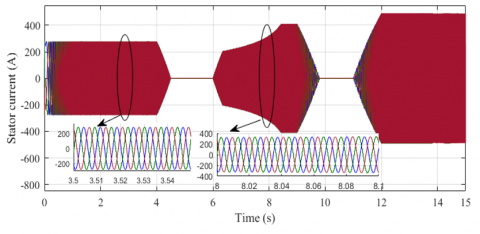Figure 17. The stator current of the PMSM

## 18.png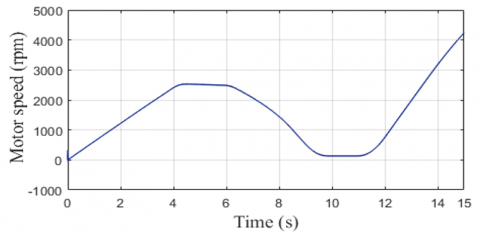Figure 18. The motor speed responses

## 19.png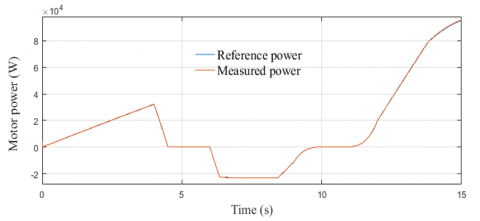Figure 19. The motor power response

## 20.pngFigure 20. The power management under different driving modes

The Figure 18 is for the motor’s speed responses; it is clear that it is a smoother version of the pedal acceleration, that comes to the fact that they are proportional. Thus, the sudden changes in the pedal are translated into a gentle continuous slope. The motor’s power is represented in the Figure 19, the measured power follows its reference with a good performance.

The Figure 20 shows the power management applied to the electric vehicle; it is a way to validate the theory of the previous section. It is undeniable that the simulation results correspond to the before mentioned theoretical characteristics and the different driving modes can be seen clearly. For example, at t=0s is the low power generation mode; the battery feeds the motor alone till t=0.4s when the fuel cell starts to work properly. From t=4s to t=6s is the medium power generation mode, the fuel cell supplies the motor, while charging the battery. At t=6s the regenerative breaking mode starts, and here the motor acts as generator and starts to charge the battery. At t=9.5s the motor finally stops, the power is non-existent in all of the components. It’s at t=11s when the high power generation modes is ON and here the fuel cell and the battery work hand in hand to feed the motor. Not that at any time t the load power is insured by summing both of the fuel cell power and the battery power.

7. Conclusions

The current work focuses on improving the performance of an EV by introducing a backstepping control reinforced with a Field oriented vector technique to control a PMSM fed by a Z-source inverter. Simulation results indicate that the backstepping control reduces current ripple and ensures reference tracking even with rapidly varying dynamics which are influenced by changing accelerator pedal position and using diverse road profiles. The energy management strategy was also applied to the system to ensure the optimal power flow in all of the components, the result of this integration have shown a good agreement between the experimental results and the theoretical characteristics; where the sum of the powers supplied by the FC and the battery pack provides the power required by the traction system while respecting the energy source constraints. The electromagnetic torque’s response is very impressive even for the sudden changes in the reference torque. The proposed model is simple and can be implemented easily with DSP or Dspace platform.

Nomenclature
 ${{v}_{sd}},{{v}_{sq}}$ The d, q components of the stator voltage ${{v}_{rd}},{{v}_{rd}}$ The d, q components of the rotor voltage ${{i}_{sd}},{{i}_{sq}}$ The d, q components of the stator current ${{i}_{rd}},{{i}_{rd}}$ The d, q components of the rotor current ${{\psi }_{sd}},{{\psi }_{sq}}$ The d, q components of the stator flux ${{\psi }_{rd}},{{\psi }_{rd}}$ The d, q components of the rotor flux T Time period ${{v}_{L}},{{v}_{C}}$ The voltage across the inductor and capacitor ${{v}_{i}}$ Inverter voltage ${{\hat{v}}_{i}}$ The peak ac voltage ${{i}_{L}},{{i}_{C}}$ Inductor and capacitor currents ${{i}_{i}}$ Inverter current V Lyapunov candidate function EV Electric Vehicle FC Fuel Cell PMSM Permanent Magnet Synchronous Motor AC Alternatif Current DC Direct Current DSP Digital Signal Processor ST Shoot-Through states NST NST states EMI Electromagnetic interference ZSI Z-source inverter VSI Voltage Source Inverter PWM Pulse Width Modulation ${{i}_{dc}}$ Dc bus current [A] C DC link capacitor [mF] ${{R}_{s}}$ Load resistance [Ω] p Number of pole pairs in a generator ${{T}_{em}}$ Electromagnetic torque [Nm] Tm The mechanical torque [Nm] Ts Sampling time [μs] f Grid frequency [Hz] $\omega$ The turbine rotation speed [rad/s] $\theta$ Angle position ${{v}_{dc}}$ The peak DC-link voltage across the inverter bridge ${{v}_{d}}$ The voltage across the diode ${{\omega }_{sl}}={{\omega }_{s}}-{{\omega }_{r}}$ The slip angular speed ${{v}_{dc}}$ Dc bus voltage [V] $v_{dc}^{*}$ Reference Dc bus voltage [V] ${{v}_{0}}$ Dc source voltage M The modulation ratio commonly used for the conventional VSI modulation B The boost factor resulting from the shoot-through zero state Rs,Rr The stator and rotor resistance ${{L}_{s}},{{L}_{r}}$ The stator and rotor inductance ${{\omega }_{s}},{{\omega }_{r}}$ The synchronous and rotor angular speed
Appendix

Table 2. PMSM parameters

 Components Rating values Rated power 57 kW Stator resistance Rs=0.0083Ω Grid frequency f=50 Hz Inductance Ld=1,741*10-4 H, Lq=2,92 *10-4 H Flux induced by magnets $\psi_{f}$ =0.0711151 Wb Number of pole pairs p=4

Table 3. Battery and fuel cell parameters

 Components Rating values Battery parameters Nominal Voltage Vbat==288 V Capacity Cn=678.260 Ah Internal resistance R=0.00384 Ω Fuel Cell parameters Fuel cell resistance Rm=0.017572 Ω Nerst voltage of one cell $E_{\text {nerst}}$ =1.1729 V Stack power nominal P=85.5 kW Stack power maximal P=85.5 kW
References

 Blaabjerg, F., Iov, F., Teodorescu, R., Chen, Z. (2006). Power electronics in renewable energy systems. 2006 12th International Power Electronics and Motion Control Conference, Portoroz, pp. 1-17. https://doi.org/10.1109/EPEPEMC.2006.4778368

 Amin, M., Arafat, Y., Lundberg, S., Mangold, S. (2011). An efficient appliance for low voltage DC house. IEEE Electrical Power and Energy Conference, Winnipeg, MB, pp. 334-339. https://doi.org/10.1109/EPEC.2011.6070221

 Noon, J., Song, H., Wen, B., Burgos, R., Cvetkovic, I., Boroyevich, D., Srdic, S., Pammer, G. (2020). A power hardware-in-the-loop testbench for aerospace applications. 2020 IEEE Applied Power Electronics Conference and Exposition (APEC), New Orleans, LA, USA, pp. 2884-2891. https://doi.org/10.1109/APEC39645.2020.9124299

 Evuri, G.R., Gorantla, S.R., Reddy, T.R.S. (2017). Enhancing the efficiency of a DC-DC converter used for hybrid electrical vehicles to suit uphill and downhill terrains. European Journal of Electrical Engineering, 19(1-2): 75-89. https://doi.org/10.3166/EJEE.19.75-89

 Peng, F.Z., Shen, M., Holland, K. (2007). Application of Z-source inverter for traction drive of fuel cell—battery hybrid electric vehicles. in IEEE Transactions on Power Electronics, 22(3): 1054-1061. https://doi.org/10.1109/TPEL.2007.897123

 Roshankumar, P., Rajeevan, P.P., Mathew, K., Gopakumar, K., Leon, J.I., Franquelo, L.G. (2012). A five-level inverter topology with single-DC supply by cascading a flying capacitor inverter and an H-bridge. in IEEE Transactions on Power Electronics, 27(8): 3505-3512. https://doi.org/10.1109/TPEL.2012.2185714

 Berkani, A., Negadi, K., Allaoui, T., Mezouar, A., Denai, M. (2019). Imposed switching frequency direct torque control of induction machine using five level flying capacitors inverter. European Journal of Electrical Engineering, 21(2): 241-284. https://doi.org/10.18280/ejee.210217

 Shanono, I.H., Nor Rul, H., Abdullah, A.M. (2018). A survey of multilevel voltage source inverter topologies, controls, and applications. International Journal of Power Electronics and Drive Systems, 9(3): 1186-1201. https://doi.org/10.11591/ijpeds.v9.i3.pp1186-1201

 Siwakoti, Y.P., Peng, F.Z., Blaabjerg, F., Loh, P.C., Town, G.E. (2015). Impedance-source networks for electric power conversion part I: A topological review. in IEEE Transactions on Power Electronics, 30(2): 699-716. https://doi.org/10.1109/TPEL.2014.2313746

 Zhu, Z.Q., Howe, D. (2007). Electrical machines and drives for electric, hybrid, and fuel cell vehicles. in Proceedings of the IEEE, 95(4): 746-765. https://doi.org/10.1109/JPROC.2006.892482

 Liu, P., Liu, H.P. (2011). Application of Z-source inverter for permanent-magnet synchronous motor drive system for electric vehicles. Procedia Engineering, 15: 309-314. https://doi.org/10.1016/j.proeng.2011.08.060

 Karabacak, K., Eskikurt, I.H. (2011). Speed and current regulation of a permanent magnet synchronous motor via nonlinear and adaptive backstepping control. Mathematical and Computer Modelling, 53(9-10): 2015-2030. https://doi.org/10.1016/j.mcm.2011.01.039

 Bouradi, S., Araria, R., Negadi, K., Marignetti, F. (2020). Nonlinear control of permanent magnet synchronous motor for high performances electric vehicle. TECNICA ITALIANA-Italian Journal of Engineering Science, 64(2-4): 317-324. https://doi.org/10.18280/ti-ijes.642-429

 Araria, R., Negadi, K., Marignetti, F. (2019). Design and analysis of the speed and torque control of IM with DTC based ANN strategy for electric vehicle application. TECNICA ITALIANA-Italian Journal of Engineering Science, 63(2-4): 181-188. https://doi.org/10.18280/ti-ijes.632-410

 Loh, P.C., Blaabjerg, F. (2013). Magnetically coupled impedance-source inverters. in IEEE Transactions on Industry Applications, 49(5): 2177-2187. https://doi.org/10.1109/TIA.2013.2262032

 Vino mol, D.V., Suji Garland, P. (2018). Three phase Z source inverter for BLDC motor using pulse width modulation technique. Proceedings of International Conference on Energy Efficient Technologies for Sustainability, St.Xavier's Catholic College of Engineering, TamilNadu, India.

 Loh, P.C., Vilathgamuwa, D.M., Lai, Y.S., Chua, G.T., Li, Y. (2005). Pulse-width modulation of Z-source inverters. in IEEE Transactions on Power Electronics, 20(6): 1346-1355. https://doi.org/10.1109/TPEL.2005.857543

 Fang, Z.P. (2003). Z-source inverter. in IEEE Transactions on Industry Applications, 39(2): 504-510. https://doi.org/10.1109/TIA.2003.808920

 Bakar, M.S., Rahim, N.A., Ghazali, K.H., Hanafi, A.H.M. (2011). Z-source inverter pulse width modulation: A survey. International Conference on Electrical, Control and Computer Engineering 2011 (InECCE), Pahang, Malaysia, pp. 313-316. https://doi.org/10.1109/INECCE.2011.5953898

 Hanif, M., Basu, M., Gaughan, K. (2011). Understanding the operation of a Z-source inverter for photovoltaic application with a design example. IET Power Electron., 4(3): 278-287. https://doi.org/10.1049/iet-pel.2009.0176

 Shen, M., Peng, F.Z. (2008). Operation modes and characteristics of the Z-source inverter with small inductance or low power factor. IEEE Transactions on Industrial Electronics, 55(1): 89-96. https://doi.org/10.1109/TIE.2007.909063

 Shen, M., Peng, F.Z. (2008). Operation modes and characteristics of the z-source inverter with small inductance or low power factor. in IEEE Transactions on Industrial Electronics, 55(1): 89-96. https://doi.org/10.1109/TIE.2007.909063

 Li, S., Zhou, M., Yu, X. (2013). Design and implementation of terminal sliding mode control method for PMSM speed regulation system. IEEE Transactions on Industrial Informatics, 9(4): 1879-1891. https://doi.org/10.1109/TII.2012.2226896

 Nadji, S., Benaicha, S. (2017). Robust integral backstepping control of PMSM for electric vehicle application. 2017 5th International Conference on Electrical Engineering - Boumerdes (ICEE-B), Boumerdes, pp. 1-6. https://doi.org/10.1109/ICEE-B.2017.8192176

 Idrissi, Z.E., Fadil, H.E., Giri, F. (2018). Nonlinear control of salient-pole PMSM for electric vehicles traction. 2018 19th IEEE Mediterranean Electrotechnical Conference (MELECON), Marrakech, pp. 231-236. https://doi.org/10.1109/MELCON.2018.8379099

 Merzoug, M.S., Benalla, H. (2010). Nonlineare backstepping control of Permanent Magnet Synchronous Motor (PMSM). International Journal of System Control, 1(1): 30-34.

 Chen, C.X., Xie, Y.X., Lan, Y.H. (2015). Backstepping control of speed sensorless permanent magnet synchronous motor based on slide model observer. International Journal of Automation and Computing, 12(2): 149-155. https://doi.org/10.1007/s11633-015-0881-2

 Matsumoto, T., Watanabe, N., Sugiura, H., Ishikawa, T. (2002). Development of fuel-cell hybrid vehicle. SAE Technical Paper. https://doi.org/10.4271/2002-01-0096